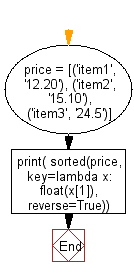﻿ Python: Sort a tuple by its float element - w3resource# Python Exercise: Sort a tuple by its float element

## Python tuple: Exercise-23 with Solution

Write a Python program to sort a tuple by its float element.

Sample Solution:-

Python Code:

``````price = [('item1', '12.20'), ('item2', '15.10'), ('item3', '24.5')]
print( sorted(price, key=lambda x: float(x), reverse=True))
```
```

Sample Output:

```[('item3', '24.5'), ('item2', '15.10'), ('item1', '12.20')]
```

Pictorial Presentation:Flowchart:## Visualize Python code execution:

The following tool visualize what the computer is doing step-by-step as it executes the said program:

Python Code Editor:

Have another way to solve this solution? Contribute your code (and comments) through Disqus.

What is the difficulty level of this exercise?

Test your Programming skills with w3resource's quiz.

﻿

## Python: Tips of the Day

Decapitalizes the first letter of a string:

Example:

```def tips_decapitalize(s, upper_rest=False):
return s[:1].lower() + (s[1:].upper() if upper_rest else s[1:])
print(tips_decapitalize('PythonTips'))
print(tips_decapitalize('PythonTips', True))
```

Output:

```pythonTips
pYTHONTIPS
```